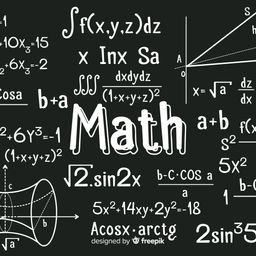Our Discord hit 10K members! 🎉 Meet students and ask top educators your questions.Join Here!Numerade Educator

# Each integral represents the volume of a solid.Describe the solid.$$\text { (a) }\pi \int_{2}^{5} y d y \quad \text { (b) } \pi \int_{0}^{\pi / 2}\left[(1+\cos x)^{2}-1^{2}\right] d x$$

## $\frac{\pi+1}{4}$

#### Topics

Applications of Integration

### Discussion

You must be signed in to discuss.
##### Top Calculus 2 / BC Educators##### Catherine R.

Missouri State University### Video Transcript

Okay, so for part eight of this were asked Thio, Um, describe the solid. So if we have the volume equals pi from 5 to 2 of y d y. So we have to realize that this is going to be using a disk method. So if you recall, um, in order to rewrite this into the disk, we would have toe have, um, pie of 5 to 2. We would need to have something squared. So what can we do to why? To get why, By squaring it. Well, we do the square root of Why So what this tells us is that for X, um, X is going to be between the square root of why and zero, because obviously, skirt of why can't really be below zero. And then why is going to be between five and two? Okay, so then four b, we have the integral from pi over two and zero. Volume equals pi, and then we have one plus cosine X squared minus one squared D X. So what this tells us is that X is between pi over two and zero and that one of our wise our first radius is one plus coastline X, and our other one is#### Topics

Applications of Integration

##### Top Calculus 2 / BC Educators##### Catherine R.

Missouri State University